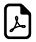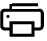Ten Times Tables Tricks10 Times Multiplication Table Trick

An online ten times table tricks.Easy way to learn 10 times table.Ten Times Table Simple Learningcan write to
can write to
can write to
can write to
can write to
can write to
can write to
can write to
can write to
can write to
can write to

How 10 times table works?

When you multiply a number by 10, put a zero on then end of it.This is the easiest Times Table.

Think 10 times.Think Zero !!!

What math times time do you want to generate ?Times Table Tricks 2 to 11►

2 Times Table Trick

4 Times Table Trick

5 Times Table Trick

6 & 7 Times Table Trick

8 Times Table TrickTop Calculators ►

Tax Calculator, Calories Burned Calculator, PERT, SD Calculator, Dog Age Calculator, Children Equal Playing Time Calculator